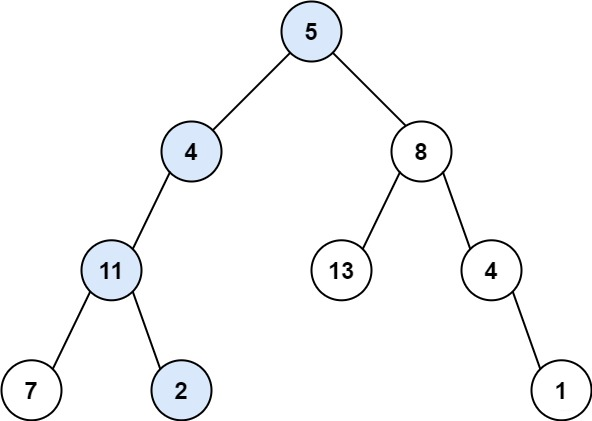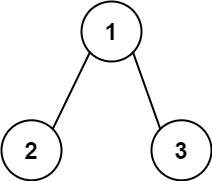# 經典筆試題: 二叉樹中和為某一值的路徑(路徑總和)

## 路徑總和``````輸入：root = [5,4,8,11,null,13,4,7,2,null,null,null,1], targetSum = 22

````````````輸入：root = [1,2,3], targetSum = 5

``````

``````輸入：root = [1,2], targetSum = 0

``````

-1000 <= Node.val <= 1000
-1000 <= targetSum <= 1000

``````/**
* Definition for a binary tree node.
* public class TreeNode {
*     int val;
*     TreeNode left;
*     TreeNode right;
*     TreeNode() {}
*     TreeNode(int val) { this.val = val; }
*     TreeNode(int val, TreeNode left, TreeNode right) {
*         this.val = val;
*         this.left = left;
*         this.right = right;
*     }
* }
*/
class Solution {

public boolean hasPathSum(TreeNode root, int targetSum) {

if(root==null)
return false;
int value=targetSum-root.val;
if(root.left==null&&root.right==null)
{

if(value==0)
return true;
}
else {

return hasPathSum(root.left,value)||hasPathSum(root.right, value);
}
return false;
}
}
``````

## 路徑總和Ⅱ

``````給定如下二叉樹，以及目標和 sum = 22，

5
/ \
4   8
/   / \
11  13  4
/  \    / \
7    2  5   1

[
[5,4,11,2],
[5,8,4,5]
]
``````

``````/**
* Definition for a binary tree node.
* public class TreeNode {
*     int val;
*     TreeNode left;
*     TreeNode right;
*     TreeNode() {}
*     TreeNode(int val) { this.val = val; }
*     TreeNode(int val, TreeNode left, TreeNode right) {
*         this.val = val;
*         this.left = left;
*         this.right = right;
*     }
* }
*/
class Solution {

List<Integer> team = new ArrayList<Integer>();
List<List<Integer>> value = new ArrayList<List<Integer>>();
public List<List<Integer>> pathSum(TreeNode root, int targetSum) {

if (root == null)
return value;
targetSum-=root.val;
if(targetSum==0&&root.left==null&&root.right==null)
{

}
pathSum(root.left, targetSum);
pathSum(root.right, targetSum);
team.remove(team.size()-1);
return value;
}
}
``````

1. star支援一下， 您的肯定是我在平臺創作的源源動力。

2. 微信搜尋「bigsai」，關注我的公眾號，不僅免費送你電子書，我還會第一時間在公眾號分享知識技術。加我還可拉你進力扣打卡群一起打卡LeetCode。「其他文章」